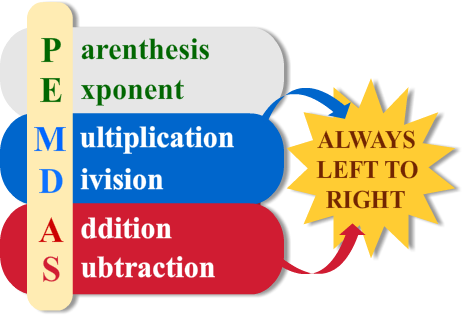# Order of Operations

Print Rate 0 stars Common Core
Lesson size:
Message preview:
Someone you know has shared lesson with you:

To play this lesson, click on the link below:

https://www.turtlediary.com/lesson/order-of-operations.html

Hope you have a good experience with this site and recommend to your friends too.

Login to rate activities and track progress.
Login to rate activities and track progress.

What is the answer to this statement?

2 + 3 x 5

2 + 3 x 5
2 + 3 x 5
5 x 5
2 + 15
25
17

The same statement can give two different answers, mathematicians agreed that everyone would use the same order when solving problems.

When solving a problem, you must always follow this order:

• Do anything inside parenthesis
• Solve any exponents.
• Multiply and divide from left to right.
• Add and subtract from left to right.Please Excuse My Dear Aunt Sally

Remember: Multiplication and division rank equally (go left to right). And same goes for addition and subtraction.

Use of Parenthesis :

• They act like a grouping symbol, not when they are representing multiplication.
• When a parenthesis is written right next to a number or variable with nothing in between, this means multiplication should be done in step 3.
• If there is any symbol in between, it is a grouping symbol and must be done first.

For example, 8(4) means to multiply 8 and 4 since there is nothing between 8 and the parenthesis.

But there is no multiplication in 6 + (4 - 1). Since there is a '+' sign in between 6 and the parenthesis, the parenthesis are a grouping symbol, so subtract '4 - 1' first.

Let's illustrate through an example used above.

2 + 3 x 5

2 + 3 x 5
2 + 3 x 5
= 5 x 5
= 2 + 15
= 25
= 17

17 , because, according to order of operations, we must multiply 3 x 5 before we add 2.

If someone wants to add 2 + 3 first, how would they do that?

Well, they'd have to add parenthesis and it would look like this:

(2 + 3) x 5
= 5 x 5
= 25

Then, the answer would be 25.

Find the answer using order of operations.
• 18 - 2 x 3

Using the order of operations, solve the expression.

18 - 2 x 3
[First, multiply 2 and 3]

18 - 6
[Now subtract 6 from 18]

12

So, 18 - 2 x 3 = 12.

• 29 - (2 + 6)

Using the order of operations, solve the expression.

29 - (2 + 6)
[First, solve the operation in parenthesis]

29 - 8
[Now subtract 8 from 29]

21

So, 29 - (2 + 6) = 21.

• 7 - 18 ÷ 9 + 1

Using the order of operations, solve the expression.

7 - 18 ÷ 9 + 1
[First, divide 18 by 9]

7 - 2 + 1
[Now subtract 2 from 7]

5 + 1

6

So, 7 - 18 ÷ 9 + 1 = 6.

• 16 ÷ 8 x 3 - 1

Using the order of operations, solve the expression.

16 ÷ 8 x 3 - 1
[First, divide 16 by 8]

2 x 3 - 1
[Now multiply 2 and 3]

6 - 1
[Now subtract 1 from 6]

So, 16 ÷ 8 x 3 - 1 = 5.

## Order of Operations

• We use PEMDAS to solve order of operations.
• P : stands for parenthesis
• E : stands for exponents
• M : stands for multiplication
• D : stands for division
• A : stands for addition
• S : stands for subtraction
• Use "Please Excuse My Dear Aunt Sally" to remember the order PEMDAS.
• Multiplication and division rank equally (got left to right). And same goes for addition and subtraction.

## Similar Lessons

Become premium member to get unlimited access.